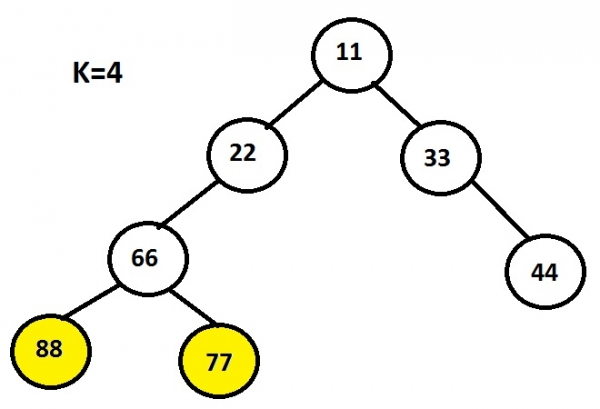# Print Leaf Nodes at a given Level in C language

The task involves printing leaf nodes of a binary tree at given level k which is specified by the user.

Leaf nodes are the end nodes whose left and right pointer is NULL which means that particular node is not a parent node.

## Example

Input : 11 22 33 66 44 88 77
Output : 88 77Here, k represents the level of a tree which needs to be printed. The approach used here is traversing every node and checking whether the node is having any pointer. Even if there is one pointer that means either left or right or both than that particular node can’t be a leaf node.

Traversing each node recursively using level order technique in which the nodes are traversed level wise starting from Left → root → right.

The below code shows the c implementation of the algorithm given

## Algorithm

START
Step 1 -> create node variable of type structure
Declare int data
Declare pointer of type node using *left, *right
Step 2 -> create function for inserting node with parameter as new_data
Declare temp variable of node using malloc
Set temp->data = new_data
Set temp->left = temp->right = NULL
return temp
Step 3 -> declare Function void leaf(struct node* root, int level)
IF root = NULL
Exit
End
IF level = 1
IF root->left == NULL && root->right == NULL
Print root->data
End
End
ELSE IF level>1
Call leaf(root->left, level - 1)
Call leaf(root->right, level - 1)
End
Step 4-> In main()
Set level = 4
Call New passing value user want to insert as struct node* root = New(1)
Call leaf(root,level)
STOP

## Example

include<stdio.h>
#include<stdlib.h>
//structre of a node defined
struct node {
struct node* left;
struct node* right;
int data;
};
//structure to create a new node
struct node* New(int data) {
struct node* temp = (struct node*)malloc(sizeof(struct node));
temp->data = data;
temp->left = NULL;
temp->right = NULL;
return temp;
}
//function to found leaf node
void leaf(struct node* root, int level) {
if (root == NULL)
return;
if (level == 1) {
if (root->left == NULL && root->right == NULL)
printf("%d",root->data);
} else if (level > 1) {
leaf(root->left, level - 1);
leaf(root->right, level - 1);
}
}
int main() {
printf("leaf nodes are: ");
struct node* root = New(11);
root->left = New(22);
root->right = New(33);
root->left->left = New(66);
root->right->right = New(44);
root->left->left->left = New(88);
root->left->left->right = New(77);
int level = 4;
leaf(root, level);
return 0;
}

## Output

If we run above program then it will generate following output.

leaf nodes are: 88 77